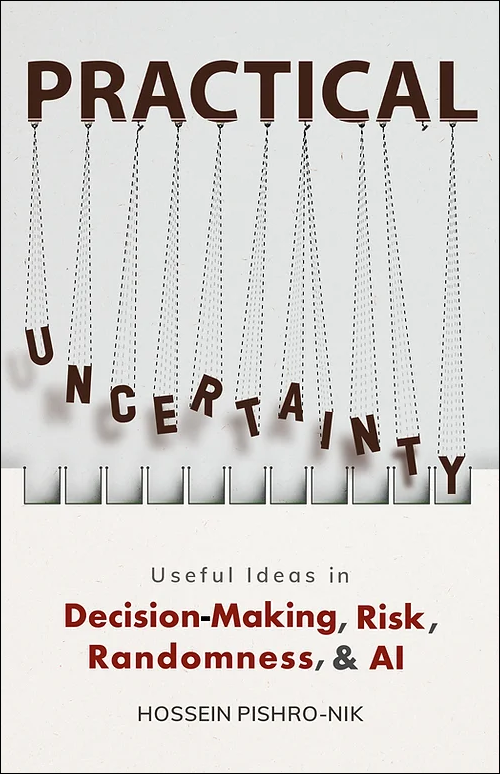## 1.4.2 Law of Total Probability

Let us start this section by asking a very simple question: In a certain country there are three provinces, call them $B_1$, $B_2$, and $B_3$ (i.e., the country is partitioned into three disjoint sets $B_1$, $B_2$, and $B_3$). We are interested in the total forest area in the country. Suppose that we know that the forest area in $B_1$, $B_2$, and $B_3$ are $100km^2$, $50km^2$, and $150km^2$, respectively. What is the total forest area in the country? If your answer is $$100km^2+50km^2+150km^2=300km^2,$$ you are right. That is, you can simply add forest areas in each province (partition) to obtain the forest area in the whole country. This is the idea behind the law of total probability, in which the area of forest is replaced by probability of an event $A$. In particular, if you want to find $P(A)$, you can look at a partition of $S$, and add the amount of probability of $A$ that falls in each partition. We have already seen the special case where the partition is $B$ and $B^c$: we saw that for any two events $A$ and $B$, $$P(A)=P(A \cap B)+P(A \cap B^c)$$ and using the definition of conditional probability, $P(A \cap B)=P(A|B)P(B)$, we can write $$P(A)=P(A|B)P(B)+P(A|B^c)P(B^c).$$ We can state a more general version of this formula which applies to a general partition of the sample space $S$.

Law of Total Probability:
If $B_1, B_2, B_3,\cdots$ is a partition of the sample space $S$, then for any event $A$ we have $$P(A)=\sum_{i} P(A \cap B_i)=\sum_{i} P(A | B_i) P(B_i).$$

Using a Venn diagram, we can pictorially see the idea behind the law of total probability. In Figure 1.24, we have $$A_1=A \cap B_1,$$ $$A_2=A \cap B_2,$$ $$A_3=A \cap B_3.$$ As it can be seen from the figure, $A_1$, $A_2$, and $A_3$ form a partition of the set $A$, and thus by the third axiom of probability $$P(A)=P(A_1)+P(A_2)+P(A_3).$$

Here is a proof of the law of total probability using probability axioms:

Proof

Since $B_1, B_2, B_3,\cdots$ is a partition of the sample space $S$, we can write

 $S$ $=\bigcup_{i} B_i$ $A$ $= A \cap S$ $=A \cap (\bigcup_{i} B_i)$ $=\bigcup_{i} (A \cap B_i) \hspace{20pt} \hspace{20pt}$ by the distributive law (Theorem 1.2).

Now note that the sets $A \cap B_i$ are disjoint (since the $B_i$'s are disjoint). Thus, by the third probability axiom, $$P(A)=P\bigg(\bigcup_i (A \cap B_i)\bigg)=\sum_{i} P(A \cap B_i)=\sum_{i} P(A | B_i) P(B_i).$$

Here is a typical scenario in which we use the law of total probability. We are interested in finding the probability of an event $A$, but we don't know how to find $P(A)$ directly. Instead, we know the conditional probability of $A$ given some events $B_i$, where the $B_i$'s form a partition of the sample space. Thus, we will be able to find $P(A)$ using the law of total probability, $P(A)=\sum_{i} P(A | B_i) P(B_i)$.

Example

I have three bags that each contain $100$ marbles:

• Bag 1 has $75$ red and $25$ blue marbles;
• Bag 2 has $60$ red and $40$ blue marbles;
• Bag 3 has $45$ red and $55$ blue marbles.
I choose one of the bags at random and then pick a marble from the chosen bag, also at random. What is the probability that the chosen marble is red?

• Solution
• Let $R$ be the event that the chosen marble is red. Let $B_i$ be the event that I choose Bag $i$. We already know that $$P(R|B_1) =0.75,$$ $$P(R|B_2) =0.60,$$ $$P(R|B_3) =0.45$$ We choose our partition as $B_1, B_2, B_3$. Note that this is a valid partition because, firstly, the $B_i$'s are disjoint (only one of them can happen), and secondly, because their union is the entire sample space as one of the bags will be chosen for sure, i.e., $P(B_1 \cup B_2 \cup B_3)=1$. Using the law of total probability, we can write

 $P(R)$ $= P(R|B_1)P(B_1)+ P(R|B_2)P(B_2)+ P(R|B_3)P(B_3)$ $=(0.75)\frac{1}{3}+(0.60)\frac{1}{3}+(0.45)\frac{1}{3}$ $=0.60$

 The print version of the book is available on Amazon.Practical uncertainty: Userful Ideas in Decision-Making, Risk, Randomness, & AI